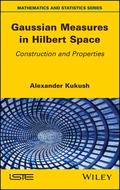John Wiley & Sons Gaussian Measures in Hilbert SpaceAt the nexus of probability theory, geometry and statistics, a Gaussian measure is constructed on a .. Product #: 978-1-78630-267-0 Regular price: \$126.17 \$126.17 In Stock

# Gaussian Measures in Hilbert Space

## Construction and Properties1. Edition January 2020
272 Pages, Hardcover
Wiley & Sons Ltd

ISBN: 978-1-78630-267-0
John Wiley & Sons

### Price: 135,00 €

Price incl. VAT, excl. Shipping

At the nexus of probability theory, geometry and statistics, a Gaussian measure is constructed on a Hilbert space in two ways: as a product measure and via a characteristic functional based on Minlos-Sazonov theorem. As such, it can be utilized for obtaining results for topological vector spaces.Gaussian Measures contains the proof for Fernique s theorem and its relation to exponential moments in Banach space. Furthermore, the fundamental Feldman-Hajek dichotomy for Gaussian measures in Hilbert space is investigated. Applications in statistics are also outlined.In addition to chapters devoted to measure theory, this book highlights problems related to Gaussian measures in Hilbert and Banach spaces. Borel probability measures are also addressed, with properties of characteristic functionals examined and a proof given based on the classical Banach Steinhaus theorem.Gaussian Measures is suitable for graduate students, plus advanced undergraduate students in mathematics and statistics. It is also of interest to students in related fields from other disciplines. Results are presented as lemmas, theorems and corollaries, while all statements are proven.Each subsection ends with teaching problems, and a separate chapter contains detailed solutions to all the problems. With its student-tested approach, this book is a superb introduction to the theory of Gaussian measures on infinite-dimensional spaces.

1. Gaussian measure in Euclidean space.
2. Gaussian measure in l2 as a product measure.
3. Borel measure in Hilbert Space .
4. Construction of Measure by its Characteristic Functional.
5.Gaussian Measure of General Form.
6. Equivalence and Singularity of Gaussian measures.7. Solutions.
Alexander Kukush is a Professor at Taras Shevchenko National University of Kyiv, Ukraine, where he teaches within its Faculty of Mechanics and Mathematics, and Department of Mathematical Analysis.